Home > CC4 > Chapter A > Lesson A.1.2 > ProblemA-21

A-21.

For each expression below, first indicate how many terms it contains. Then copy and simplify each expression by combining like terms. Algebra tiles may be helpful. Homework Help ✎

1. 2x − 3x − 3 + 4x2 + 10 + x

2. −4x + y2 − 9 + 10 − x + 3x − 4y2

3. 2x2 + 30y + 3x2 + 4x2yx

4. 0 − 20 − 5xy + 4y2 + 10 − y + xy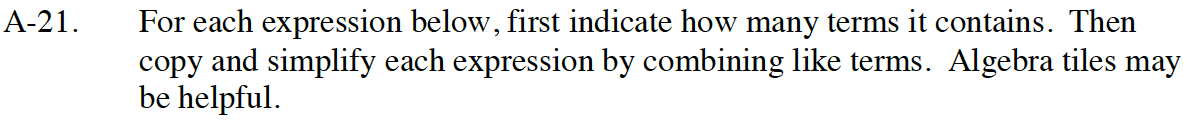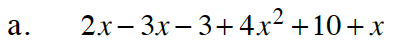4x2 + (2x − 3x + x) + (10 − 3)

4x2 + 7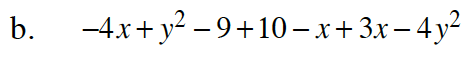It may helpful to rearrange the terms so that all like terms are next to one another before simplifying.
Remember to double-check your work.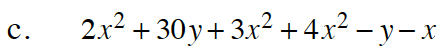Try thinking of these as algebra tiles.
Can you combine x2-tiles with x-tiles or unit tiles?
If it helps you, you can use algebra tiles to help you solve this problem.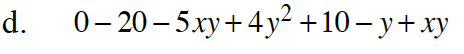Follow the steps outlined in part (a) and the hints from parts (b) and (c).

4y2 − 4xyy − 10